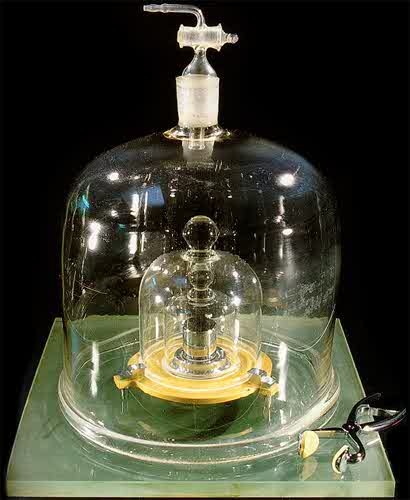# Significant Figures: Basic skills

This post covers the basic skills you need to know in order to correctly specify the number of significant figures in a calculation. A work sheet is attached for homework. Continue reading Significant Figures: Basic skills

# Systems of Units

What are Units of Measure?
What is meant by a System of Units?

Units of measure are names we give to specific items, sizes, lengths, volumes, weights, masses, temperatures, periods of time etc. Most units of measure were developed by scientists and engineers for convenience. The units we use today were agreed on by people across an industry or a science. Some units are related directly to physical phenomenon. These “physical” constants include the speed of light “c”, and the size of an atomic nucleus, the “barn” because scientists were surprised how large the nucleus was when they first  measured it and someone jokingly said “It’s as big as a barn!”

Base units are units which cannot be derived from combinations of other units. Derived units are derived from combinations of base units. Base units include meters {m}, kilograms {kg}, seconds {s}, centimeters {cm}, grams {g}, inches {“}, feet {‘}, slugs {} and others which we will discover later in this course. Some derived units are Newtons {N}, Joules {J}, Watts {W}, dynes {D}, and ergs {e}, pounds {#}, and horsepower {hp}. Notice that I frequently but units in {} brackets when defining them.

A system of units is a group of units which are commonly used together. The three most important systems of units are MKS, CGS, and British or Engineering Units.

In this course, we will be using the MKS system {meters, kilograms, seconds} as the MKS system is best suited to describing large objects such as cars, trains, planets, and people. The MKS system was agreed upon and standardized and named “Système international d’unités” or SI for short. The process of standardizing the MKS system of units began in 1791 and was finally agreed to by an international treaty in 1875 by the Treaty of the Metre. The process continued into the 1950’s with the addition of 3 more base units and a large number of derived units.

### The MKS or SI System of Units

Here’s a little history of the “meter”, the “kilogram” and the “second”.

### The MeterThe idea of the meter as a unit of length started in about 1665 when the Dutch Physicist Christiaan Huygens (arguably one of the greatest minds in the history of science) observed the length of a pendulum which “ticked” with a half period of 1 second (1 second to swing from left to right, another second to swing back), had a length of 39.26 inches. Christian Huygens is also the person who developed the first pendulum clock or “Grandfather Clock” in 1656).In 1668, Wilkins and Christopher Wren proposed using the length of this pendulum as a standard of length and called the apparatus the “seconds pendulum”. But this definition of the meter did not catch on at the time.

In the 18th century, there were two approaches to the definition of the standard unit of length. One favored Wilkins approach: to define the meter in terms of the length of a pendulum which produced a half-period of one second. The other approach was to define the meter as one ten-millionth (1/10 000 000) of the length of a quadrant along the Earth’s meridian; that is, the distance from the Equator to the North Pole. This means that the quadrant (a section/distance 1⁄4 of the Earth’s circumference) would have been defined as exactly 10 000 000 metres (10 000 km). In 1791, the French Academy of Sciences selected the meridional definition over the pendular definition because the force of gravity varies slightly over the surface of the Earth, which affects the period of a pendulum.Question: Calculate the radius of the earth based on the meridional definition of the meter.

*************
``` ```
*************
The standard meter today is 40.36 inches, or approximately 1 1/3 yards.

### Mass

Now that we have the meter as a standard of length, let’s talk about volume. Volume will lead us to a definition of mass.

There is very little information available about how this started, so I’ll tell what I think happened.

In the 1700’s early chemists wanted a standard of volume and mass so they could compare the results of their research. They figured out how to make a square vessel 1/10th of a meter (10 cm, approximately 4 inches) on each side. They probably made the vessel out of slabs of polished stone or glass, and sealed along each edge with wax. Then they agreed that the amount of water contained in this vessel would be a standard of weight or mass. They called the volume of this vessel 1 liter, and they named the weight or mass of the water contained in this vessel 1 kilogram. In 1889, the CGPM (Conférence Générale des Poids et Mesures) created at standard of mass made from a Platinum – Iridium alloy (a very hard allow which could be accurately machined).Later in 1901 the CGPM clarified that this kilogram was the standard unit mass, resolving the confusion created by using weight and mass interchangeably.

# Physics Unit 0: What is Physics?

This lesson is the first lesson in a 2 semester high school physics course. In this introduction to Physics, we discuss the main areas of Physics, subtopics and why Physics is important.

# Acceleration (motion under constant acceleration) – Part 1

Acceleration Part 1: Learning Objective: average and instantaneous acceleration,  calculating distance travelled from a v vs t graph, formula to calculate distance travelled assuming constant acceleration. Contains Keynote (powerpoint) presentation. Continue reading Acceleration (motion under constant acceleration) – Part 1